# Connection form

(diff) ← Older revision | Latest revision (diff) | Newer revision → (diff)

A linear differential formon a principal fibre bundlethat takes values in the Lie algebraof the structure groupof. It is defined by a certain linear connectionon, and it determines this connection uniquely. The values of the connection formin terms of, where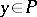and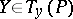, are defined as the elements ofwhich, under the action ofon, generate the second component ofrelative to the direct sum. Hereis the fibre ofthat containsandis the horizontal distribution of. The horizontal distribution, and so the connection, can be recovered from the connection formin the following way.

The Cartan–Laptev theorem. For a formonwith values into be a connection form it is necessary and sufficient that: 1)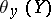, for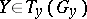, is the element ofthat generatesunder the action ofon; and 2) the-valued-formformed from, is semi-basic, or horizontal, that is,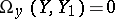if at least one of the vectors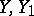belongs to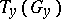. The-formis called the curvature form of the connection. If a basis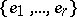is defined in, then condition 2) can locally be expressed by the equalities: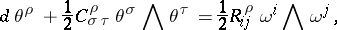where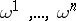are certain linearly independent semi-basic-forms. The necessity of condition 2) was established in this form by E. Cartan ; its sufficiency under the additional assumption of 1) was proved by G.F. Laptev . The equations

for the components of the connection form are called the structure equations for the connection in, the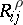define the curvature object.

As an example, letbe the space of affine frames in the tangent bundle of an-dimensional smooth manifold. Thenandare, respectively, the group and the Lie algebra of matrices of the form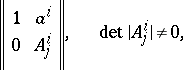and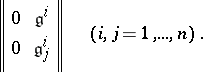By the Cartan–Laptev theorem, the-valued-formonis the connection form of a certain affine connection onif and only if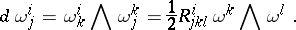Hereandform, respectively, the torsion and curvature tensors of the affine connection on. The last two equations for the components of the connection form are called the structure equations for the affine connection on.

How to Cite This Entry:
Connection form. Encyclopedia of Mathematics. URL: http://encyclopediaofmath.org/index.php?title=Connection_form&oldid=13808
This article was adapted from an original article by Ãœ. Lumiste (originator), which appeared in Encyclopedia of Mathematics - ISBN 1402006098. See original article